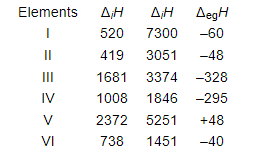# The first (ΔiH1) and the second (ΔiH) ionization enthalpies (in kJ mol–1) and the (ΔegH) electron gain enthalpyQuestion:

The first $(\Delta ; H 1)$ and the second $(\Delta, H)$ ionization enthalpies $\left(\right.$ in $\left.\mathrm{kJ} \mathrm{mol}^{-1}\right)$ and the $\left(\Delta_{\mathrm{eg}} H\right)$ electron gain enthalpy $\left(\right.$ in $\left.\mathrm{kJ} \mathrm{mol}^{-1}\right)$ of a few elements are given below:Which of the above elements is likely to be :

(a) the least reactive element.

(b) the most reactive metal.

(c) the most reactive non-metal.

(d) the least reactive non-metal.

(e) the metal which can form a stable binary halide of the formula MX2, (X=halogen).

(f) the metal which can form a predominantly stable covalent halide of the formula MX (X=halogen)?

Solution:

(a) Element $\mathrm{V}$ is likely to be the least reactive element. This is because it has the highest first ionization enthalpy $\left(\Delta_{i} \mathrm{H}_{1}\right)$ and a positive electron gain enthalpy ( $\left.\Delta_{\text {eg }} \mathrm{H}\right)$.

(b) Element II is likely to be the most reactive metal as it has the lowest first ionization enthalpy $\left(\Delta_{i} H_{1}\right)$ and a low negative electron gain enthalpy ( $\Delta_{\text {eg }} H$ ).

(c) Element III is likely to be the most reactive non-metal as it has a high first ionization enthalpy $\left(\Delta_{;} \mathrm{H}_{1}\right)$ and the highest negative electron gain enthalpy $\left(\Delta_{\mathrm{eg}} \mathrm{H}\right)$.

(d) Element $\mathrm{V}$ is likely to be the least reactive non-metal since it has a very high first ionization enthalpy $\left(\Delta_{i} \mathrm{H}_{2}\right)$ and a positive electron gain enthalpy $\left(\Delta_{\text {eg }} \mathrm{H}\right)$.

(e) Element VI has a low negative electron gain enthalpy $\left(\Delta_{\mathrm{eg}} \mathrm{H}\right)$. Thus, it is a metal. Further, it has the lowest second ionization enthalpy $\left(\Delta_{i}, \mathrm{H}_{2}\right)$. Hence, it can form a stable binary halide of the formula $\mathrm{MX}_{2}(\mathrm{X}=$ halogen $) .$

(f) Element $V$ has the highest first ionization energy and high second ionization energy. Therefore, it can form a predominantly stable covalent halide of the formula $M X(X=h$ halogen $)$.